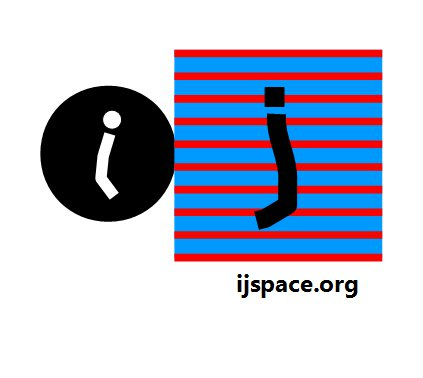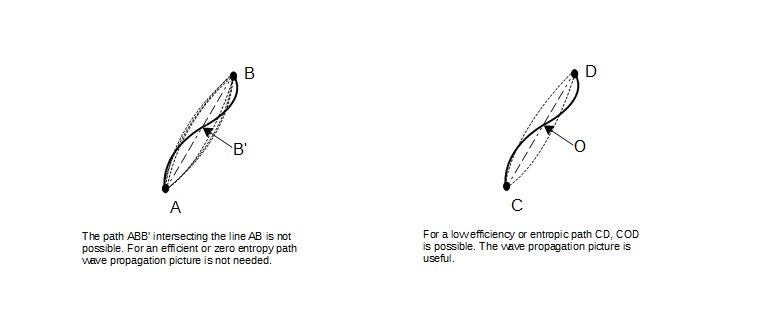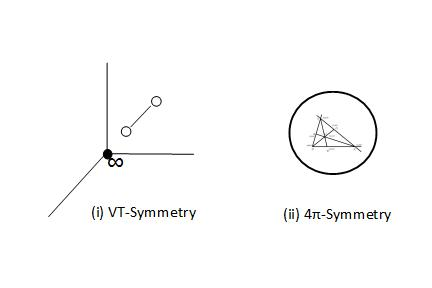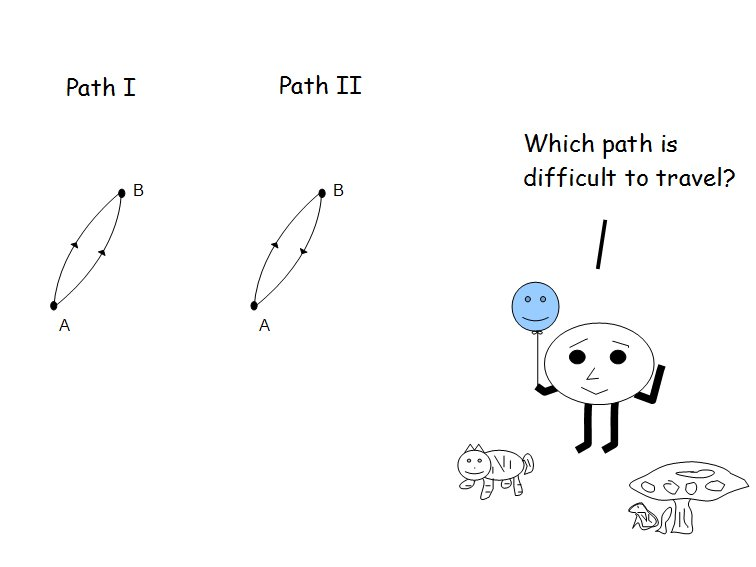#A  Paradox 15th February 2016 "...And then we might proceed, on the same plan, to a Third Construction, and to indefinitely many others following: building up thus what Professor Möbius, in his Barycentric Calculus, has proposed to call a Geometric Net in Space." - Sir William Rowan Hamilton in "ON GEOMETRICAL NETS IN SPACE", Proceedings of the Royal Irish Academy, vol. vii (1862), pp. 532-582.   "It is only in quantum theory that Newton’s differential method becomes inadequate, and indeed strict causality fails us. But the last word has not yet been said. May the spirit of Newton's method give us the power to restore unison between physical reality and the profoundest characteristics of Newton's teaching - strict causality." - Einstein as quoted in "Subtle is the Lord: The Science and the Life of Albert Einstein" by Abraham Pais.   "We regard quantum mechanics as a complete theory for which the fundamental physical and mathematical hypotheses are no longer susceptible of modification." - Heisenberg and Max Born, paper delivered to Solvay Congress of 1927. (Ref. www.aip.org) A paradox is a seemingly self-contradictory statement which may or may not be true.  In discrete measurement space, usually a paradox occurs when an observer has a mechanism which explains its own PE1 measurements, yet the same mechanism is inadequate to explain a phenomenon of higher information content.  In the later case the following condition is arrived at in the observer's measurement space, ¬∈   =   ∈.     Above statement defines a paradox in a discrete measurement space or j-space.  It will hold true for any observer with finite capacity.  Or we can also say that whatever an observer measures within its measurement-metric in j-space, will eventually lead to a paradox.     One of the earlier paradox we could imagine, would be whether the laws of physics are the same in a reference frame at rest and a reference frame moving at a constant velocity with respect to the the reference frame at rest.  Until Newton differentiated between the inertial and the non-inertial reference frames, it would be a hotly debated topic.  As it happens we are well past it.     Two of the most promising and established theories explaining physical phenomena in nature, are the General Theory of Relativity (GTR) and Quantum Mechanics (QM).  Both of them are brilliant and their predictions verified with numerous experiments.  Both theories lead to the concepts of Newton's Classical Mechanics, under limits.  Yet they seem to contradict each other.  Einstein would never agree to existence of universe and more importantly the progression of universe based solely on the randomness inherent in QM.  On the other hand question is always asked where are the gravitational waves* or the gravitons?  Then there is Maxwell's Electromagnetic Theory which seems to work very well with QM and QED.  How come we can not unify the GTR and QED?  In recent times similar debate has been ongoing between two leading contenders for unification, the String Theory and the theory of Loop Quantum Gravity.  There are so many arguments given and papers written trying to prove superiority of one theory over other, but there is always this sense of disquiet that we really do not have a complete explanation for the phenomena we observe.     Fortunately in our simple world the requirements are much simpler.  We do not wish to explain the universe nor the beautiful world of the subatomic particles.  We just wish to determine two points, A and B, nearest to each other and then given certain resources we wish to find the most efficient path(s) between A and B.  We would also like to know that if we arrived at the point B from A then is it possible to return to the point A from B?  One can not be careful enough making traveling plans in an entropy-laden universe.We know that in true j-space no two points are alike.  If they are not alike then how could they be connected by a path?  The path must have some property common to A and B, or A and B themselves would have some common property which would connect them.  The best route is where the properties of the points A and B and the path connecting them are identical1.  We are then guaranteed the maximum efficiency to arrive at the point B from A and the return to the point A from B.  It is essentially a single measurement and hence a zero-entropy solution for the observer.    We apply the VT-symmetry where both observers are identical.  These two observers represent the extrema of the "finiteness".  We can take points A and B to be these two observers2.  We recollect that we needed VT-symmetry to determine the origin of the measurement space of an observer. The problem of determination of two nearest points in an observer's metric is equivalent to determining the origin.  And the most efficient path will be determined by the observer with maximum efficiency (v/c =1).  An example is, the Fermat's principle equivalent in j-space.  We keep in mind that all these arguments are valid only within an observer's measurement metric.3We have used Hamilton's Anharmonic Coordinates to further elaborate the concept of "finiteness". We then correlate "finiteness" to the geometry, unit-point sphere, with 4π-symmetry.  The concepts of "finiteness" and "quantum" are different from each other.     The "quantum" as we know it, is based on the empirical study performed by Planck on radiation.  The uncertainty relation followed, derived by Heisenberg which correlated position and momentum to Planck's constant h.     On the other hand, the concept of "finiteness" is a requirement stemming from the observer's inability to measure origin accurately.  Thus the arguments leading to "finiteness" are more phenomenological in nature.4  If that is truly the case, then eventually we must be able to explain what we measure empirically, based on what we understand phenomenologically. We shall continue this discussion further by describing the Duality. __________________________ * Well! This got taken care of recently at LIGO. 1. Obviously we can not accomplish it without the assumption of a symmetry.  The universe a measurement itself, is a subset of pure j-space. 2. Thus we have moved from "infinitesimal" to "finiteness". 3. The measurement-metric may or may not be in Euclidean or Minkowski space. 4. We do not associate any physical constant or parameter with "finiteness".  The physical constants and parameters represent the interpretation of "finiteness" in the observer's measurement-metric. Previous Blogs:   Nutshell-2015 Chiral Symmetry Sigma-z and I Spin Matrices Rationale behind Irrational Numbers The Ubiquitous z-Axis Majorana ZFC Axioms Set Theory Nutshell-2014 Knots in j-Space Supercolliders Force Riemann Hypothesis Andromeda Nebula Infinite Fulcrum Cauchy and Gaussian Distributions Discrete Space, b-Field & Lower Mass Bound Incompleteness II The Supersymmetry The Cat in Box The Initial State and Symmetries Incompleteness I Discrete Measurement Space The Frog in Well Visual Complex Analysis The Einstein Theory of RelativityBest viewed with Internet Explorer.